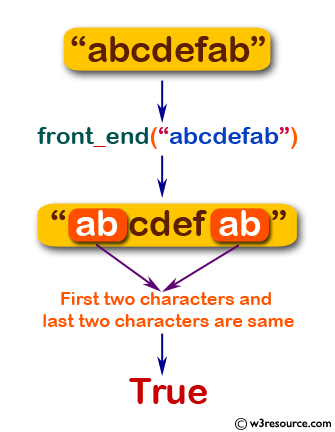﻿ Swift String Programming Exercise: Check if the first two characters are same of the last two characters of a given string - w3resource# Swift String Exercises: Check if the first two characters are same of the last two characters of a given string

## Swift String Programming: Exercise-19 with Solution

Write a Swift program to check if the first two characters are same of the last two characters of a given string.

Pictorial Presentation:Sample Solution:

Swift Code:

``````func front_end(_ a:String) -> Bool {

if String(a.characters.prefix(2)) == String(a.characters.suffix(2))
{
return true
}
else
{
return false
}
}
print(front_end("abcdefab"))
print(front_end("abcdefba"))
print(front_end(""))
```
```

Sample Output:

```
true
false
true
```

Swift Programming Code Editor:

Improve this sample solution and post your code through Disqus

What is the difficulty level of this exercise?

﻿